# Spatio-Temporal Changes in the Turbulent Kinetic Energy of a Rectangular Jet Impinging on a Slotted Plate Analyzed with High Speed 3D Tomographic-Particle Image Velocimetry

Spatio-Temporal Changes in the Turbulent Kinetic Energy of a Rectangular Jet Impinging on a Slotted Plate Analyzed with High Speed 3D Tomographic-Particle Image Velocimetry

Hassan H. Assoum* Jana Hamdi Kamel Abed-Meraïm Marwan Al Kheir Tarek Mrach Louay El Soufi Anas Sakout

Faculty of Mechanical Engineering, Beirut Arab University, Tripoli, Lebanon

School of Engineering, Lebanese American University, Byblos, Lebanon

LaSIE, University of La Rochelle, La Rochelle, France

Faculty of sciences III, Lebanese University, Tripoli, Lebanon

Corresponding Author Email:
h.assoum@bau.edu.lb
Page:
1071-1079
|
DOI:
https://doi.org/10.18280/ijht.370416
11 December 2018
|
Accepted:
25 November 2019
|
Published:
26 December 2019
| Citation

OPEN ACCESS

Abstract:

The purpose of this study is to investigate the temporal changes of the Turbulent Kinetic Energy (TKE) of an impinging rectangular self-excited jet in order to identify a reduced zone that is active in producing TKE. An experimental set up consisting of a rectangular jet impinging on a slotted plate was considered for a Reynolds Number Re=5435. This configuration is accompanied by a high level of noise due to the appearance of self-sustained tones that occur in optimal conditions for energy transfer between fluctuating velocity and acoustic field. High Speed Tomographic Particle Image Velocimetry (PIV) technic was used to evaluate the Turbulent Kinetic Energy (TKE) field derived from the aerodynamic one. Through this study, it was found that a reduced volume of height of 0.5*H and a length of 2*H (where H is the height of the nozzle exit) was satisfactory to represent the three-dimensional TKE activity between the jet exit and the plate. The findings of this research lies in investigating the evolution of the TKE that could feed the acoustic generation in order to develop new techniques of noise control. Such sub-volume of the flow would also save a considerable time of calculation.

Keywords:

acoustic comfort, self-sustained tones, indoor air quality, fluctuating velocity, noise control

1. Introduction

An impinging jet is a flow configuration in which a fluid passing through a simple geometric structure hits a wall. They are widely use in several applications such as in drilling process where a jet is employed for its effect of erosion and cleaning. In building applications, these jets are encountered in ventilation systems to improve mixing and diffusion of airflows, making it possible to provide the fresh air required for comfort in living rooms while avoiding stagnation areas and minimizing energy consumption. They could also be employed for cooling of computer CPUs  or to improve efficiency of solar air heater .

Impinging jets exhibit rich fluid-structure interaction phenomena and they are accompanied in some cases by a self-sustaining/resonating loop. Self-resonating jets take place due to a feedback loop that was described by Ho and Nosseir [3, 4]. It involves an upstream propagating pressure created by the impingement of downstream coherent structures on a surface. This upstream propagating wave forces the shear layer close to the exit of the jet to oscillate inducing a collective interaction in which born large coherent vortex rings. These large structures could be advantageous to promote cavitation and improve drilling process  where the passage of structured vortex at the wall exerts high shearing and suction forces what enhance erosivity and cleaning action . In ventilation systems, these jets become source of noise pollution in some cases through the appearance of the self-sustained sounds. These sounds occur when the flow interacts with an obstacle with holes or slots in its path; they are desirable in the case of musical instruments, but they are considered as a source of discomfort in most technical applications and was considered in many studies [7-10]. Assoum et al. investigated a rectangular jet impinging on a slotted plate and showed that in self-resonating jets, a high-level noise is created and principally distributed over well-defined frequency peaks . The pulsations of the jet could be obtained by an external source of excitation. Crow et al. described the aerodynamics of a jet excited by an external wave at a certain frequency . They found that for an optimal structuring of the jet, the frequency of the external wave could be given by a Strouhal number, $S t=\frac{f * d}{V}=0.3$, (where V is the velocity of the jet at the exit of the nozzle of diameter d). Johnson et al. present acoustic oscillators that passively accomplish pulsations in the jet instead of using an external exciter such as loudspeaker . The passive excitation could be acquired by equipping the jet nozzle with a type of acoustic chamber adjusted to resonate at a well-defined frequency or by modelling a suitable nozzle to excite the jet at the exit. Such devices are forms of "whistles" and are completely passive .

The acoustic wave that is generated in the region of impingement produce a modulation of the shear layer near the jet outlet and an amplification of the instabilities. The excitation frequency of the instabilities depends on the jet size, the Reynolds number and the distance between the jet outlet and the impinged plate. This feedback loop maximizes energy transfer from the aerodynamic field to the acoustic field and creates a source of aero-acoustic noise that can reach high levels. Indeed, Powell developed an analogy that takes into account sound sources created by vortices , and Howe was the first to use this analogy in the case of near-wall flow . The corollary of Howe's energy makes it possible to evaluate the acoustic power exchanged with the flow. This requires three quantities: the vorticity, the flow velocity and the acoustic velocity using experimental or numerical data. Thus, when the self-sustained loop is optimized, energy transfer from the aerodynamic field to the acoustic field occurs in a geometric configuration, allowing optimal interactions between the acoustic field and the aerodynamic fluctuations. Manufacturers are seeking ways to reduce or eliminate this noise. Despite its apparent simplicity, it is a difficult phenomenon to control.

Toutiaei et al.  reported the energy balance for a free jet using a contracted inlet nozzle by the mean of laser Doppler anemometry. They showed a large TKE inside the mixing layer. Many other studies have been performed from PIV techniques to estimate the TKE balance, the individual components of the Reynolds stress tensor and the turbulent dissipation rate in a turbulent round jet using stereoscopic Particle Image Velocimetry . In other studies, the TKE was considered in an impinging jet and the dissipation term from instantaneous Stereo-PIV velocity fields was calculated in order to compare swirling and non-swirling impinging jets (for Re = 8900, h/d = 3 and considering a contracted nozzle) . In numerical studies, the swirling effect of impinging jet on heat transfer over a plate having obstacles was considered . The turbulence energetics of an axisymmetric impinging jet flow in the wall jet regions was investigated by using two-dimensional PIV technique . They showed that TKE differ greatly between different regions of the jet especially in near-field and far-field wall jet regions. In large-eddy simulations, TKE balance was presented on the impingement wall where non-negligible turbulence dissipation was reported .

The TKE in impinging jets is of high importance because it evaluates the extracted energy from the mean flow, and dissipated or transferred to other fields by various ways. But few studies considered the TKE derived from High speed Tomographic-PIV velocity fields for rectangular impinging jets. This technique enables the instantaneous measurement of all three velocity components in a complete 3D measurement volume (3 Dimensions, 3 Components) what offer the possibility to have more accurate data without neglecting any dimension differently to Assoum et al. who considered only two components using 2D PIV . In this paper, we calculated the TKE derived from High speed Tomographic-PIV measurements. Our main objective is to investigate spatio-temporal changes of the TKE field, which is very important since it would provide information on changes in the main source of energy for the acoustic field that should be controlled. In section 2, we present the experimental device and the metrology of High speed Tomographic-PIV. In section 3 we plot the variation of TKE with respect to time and space for different zones of interest and we discuss the possibility for representing the TKE activity in the flow by a sub-volume near from the wall of impact. Such representation is of high importance since it allows us to identify the involved zone in feeding the acoustic field. In addition, it would save a considerable time of calculation. In section 4, the conclusions are given and perspectives are opened to the next work.

2. Experimental Setup

2.1 The rectangular impinging jet

The set-up of our study is shown in Figure 1 . The flow is created by a compressor (1), which is ordered by a Variable Frequency Drive (2) that controls the frequency of rotation and therefore the exit velocity of the flow. The airflow crosses a $1 m^{3}$ settling chamber (3) fitted with metal grids to guarantee acoustic insulation of the duct from the blower. Next, it passes through a rectangular tube (4) $1250 \mathrm{mm}$ length with a $90 \times 190 \mathrm{mm}^{2}$ cross - section, which is extended by a rectangular convergent ( 5 ). Honeycomb sections are inserted into the rectangular tube to smooth the flow and eliminate any mean swirl. Hence, the set-up creates a free jet $H=$ $10 \mathrm{mm}$ high and $L_{z}=190 \mathrm{mm}$ wide. Parallel to the convergent, a $4 \mathrm{mm}$ thick aluminum plate ( 6) is fitted with a beveled slot ( 7) with the same dimensions as the convergent outlet and perfectly aligned with it using a displacement system.

A 4 mm thick aluminum plate (6) is fitted parallel to the convergent with a beveled slot (7) with the same dimensions as the convergent outlet. Distance $L$ is from the exit of the convergent to the impinged plate ($\frac{L}{H}$=4 in this paper). The \right. Reynolds number is calculated as follows: $R e=U_{0} . H / v$ where $v$ is the kinematic viscosity of air, and $\mathrm{U}_{0}$ the initial velocity at the exit of the jet. The Reynolds number $R e=$ 5435 in this work.

## 1.png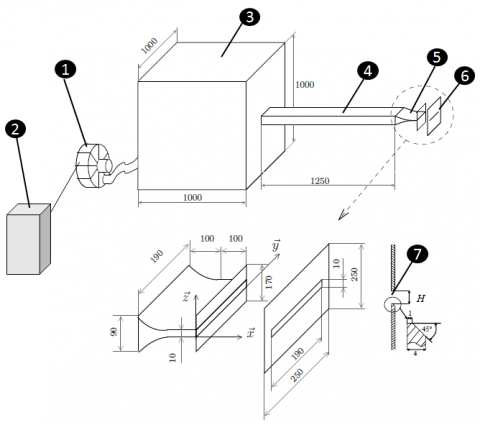Figure 1. Rectangular-impinging jet setup

2.2 Tomographic-PIV

In order to study the characteristics of an impinging jet on a slotted plate for a confinement of $\frac{L}{H}=4$ and a Reynolds number $R e=5435,$ a Tomographic-PIV system was employed in this study to measure the three velocity components projected in the volume laser.

Time resolved Tomographic-PIV consists in recording two successive and separate images of the flow (Illuminated by a laser volume as illustrated in Figure 2) and cross-correlating them to obtain a two-component kinematic field. The interval between the images “dt” is determined as a function of the initial velocity of the jet and the depth of view. High-speed flows were studied using a pulsed laser with a sufficiently low interval. In fact, by looking at a small interval, the displacement caught by the camera is small, providing a high spatial and temporal resolution of the velocity fields obtained .

A $527 \mathrm{nm}$ Nd: YLF LDY 300 Litron laser was used with an energy of $30 \mathrm{mJ}$ per pulse, extended by an $1800 \mathrm{mm}$ long laser arm. The laser arm is equipped with a volume generator that is formed by diverging lenses that generate a volume illumination. The volume measured in the present study was $60 \times 40 \times 18 \mathrm{mm}^{3}$.

## 111111.pngFigure 2. Illumination of the volume measured

Three Phantom V711 cameras with 1280 x 800 voxels were used. The cameras were positioned as follows: two cameras on the right side of the jet exit, one above the other. The third camera was placed on the left of the jet at the same level as camera 1. These three cameras were 40 cm from the measurement volume. The cameras used were set-up at an angle θ relative to the normal of the laser plane, which was chosen to optimize the quantity of light received. Moreover, they were provided by a Scheimpflug arrangement, allowing an optimization of the sharpness of the particles. The pulsed laser light has a frequency response of 10 kHz. Particle seeding was performed using a TOPAS SLG 270 condenser aerosol generator which ensures the generation of 4 μm particles. For the present measurement, the pressure was set at 4 bars, the temperature of the Saturator at 240°C and that of the reheater at 300°C. It should be noted that particle size was measured using the PAM 510 granulometer.

The views from different cameras need to be triangulated in order to obtain a tomographic reconstruction. The accuracy of the reconstruction of the 3D light distribution is required to be a small fraction of the particle size . The volume self-calibration process requires two steps: the first is the calculation of the vector map of disparities, and the second corrects the calibration. These two steps must be repeated several times until the disparity (volume error) is less than 0.1 voxel in all sub volumes. Couples of PIV images were acquired with a sampling frequency of 2 kHz. Once the particle density was optimized, the time interval $\Delta t$ between the first and the second exposure was adjusted so that the particles moved a maximum of about 7 to 10 voxels.

The three-dimensional distribution of the light scattered by the tracers (particles) was reconstructed in the form of a 3D matrix of voxels representing the luminous intensity of the object plane (physical space) from its projection onto camera sensors. This reconstruction was carried out using the MART reconstruction technique ("Multiplicative Algebraic Reconstruction Technique") which is an iterative technique, as already mentioned. The accuracy of reconstruction depends heavily on several factors. These factors include the number of cameras and the density of recorded images. The reconstruction of the kinematic field by the algorithm was achieved using the DaVis 8.3.0 software from LaVision that was used for our measurements.

The "calculation of correlation peaks" associated with the "tomographic PIV" module calculates the velocity vector field from the reconstructed volume. The reconstructed image distribution in the volume was analyzed with an iterative multi-grid volume deformation scheme, attaining a 48 voxel interrogation box size with 75% overlap, yielding the instantaneous three-dimensional velocity volume. Finally, the post-processing operation of the "Tomographic PIV" group was used to reduce the noise in the vector field.

3. Results and Discussions

3.1 Turbulent Kinetic Energy (TKE)

The TKE will be considered in this part for a Reynolds number $\mathrm{Re}=5435$ and a confinement ratio $\frac{L}{H}=4 .$ The Reynolds number was chosen based on previous work where it was shown that for this Reynolds number, there was a high acoustic level and self-sustained tones were produced . The change in TKE is important in such configurations to get a better understanding of its role in providing the acoustic field by aerodynamic energy.

The distribution of the average normalized TKE is shown in Figure 3. The coordinate origin is considered to be at the jet impingement and the y-axis is along the axis of the jet. The maximum streamwise velocity is denoted by $U_{0} .$ The volumetric TKE in Figure 3 was calculated on each voxel of the Tomographic-PIV image for a volume $(\mathrm{X}, \mathrm{Y} \text { and } \mathrm{Z})$ as follow:

$TKE=\frac{u^{\prime 2}+v^{\prime 2}+w^{\prime 2}}{2}$                     (1)

where, $u^{\prime}, v^{\prime}$ and $w^{\prime}$ are the fluctuations of velocities in $\mathrm{X}, \mathrm{Y}$ and $Z$ directions respectively. The fluctuating velocities were calculated as follow:

$u^{\prime}=U-U_{m}$                    (2)

$v^{\prime}=V-V_{m}$                     (3)

$w^{\prime}=W-W_{m}$                       (4)

where, U, V and W are the instantaneous velocities in $\mathrm{X}, \mathrm{Y}$ and $\mathrm{Z} \quad$ directions $\quad$ respectively. $\quad$ The $\quad$ terms $\mathrm{U}_{\mathrm{m}}, \mathrm{V}_{\mathrm{m}},$ and $\mathrm{W}_{\mathrm{m}}$ correspond to the mean velocities in $\mathrm{X}, \mathrm{Y}$ and Z directions, respectively. The TKE was normalized by the square of the maximum streamwise velocity $U_{0}^{2}\left(i . e . \frac{T K E}{U_{0}^{2}}\right)$

In order to identify volumes of high $\mathrm{TKE},$ the velocity measured in the volume extended from $X=0$ to $X=40 \mathrm{mm}$ from $\quad Y=-15 \mathrm{mm} \quad$ to $\quad Y=15 \mathrm{mm} \quad$ and $\quad$ from $\quad Z=-5 m m$ to $Z=5 m m$ were divided into seven sub-volumes, shown in Figure $3 .$ The dimensions in the Y-direction $(-15 m m<Y<15 m m)$ were chosen in such a way that they contained vortice deformation zones in which the acoustic source is created in the impact region.

3.2 Spatio-temporal change of the TKE

In order to plot the temporal change of the TKE obtained from the different volumes, the sum of $\frac{T K E}{U_{0}^{2}}$ over each volume was divided by the volume in which the TKE was calculated. By performing this calculation for successive TomographicPIV images, we obtained the normalized TKE per unit of volume as a function of time. The time was normalized by the period of self-sustained tones of the acoustic signal obtained for this Reynolds number, where $T=\frac{1}{f} \approx 6$ ms for $R e=$ $5435 .$ In this way, the volumetric normalized TKE per unit of volume was obtained as function of the acoustic period of the self-sustained tones.

## 3.png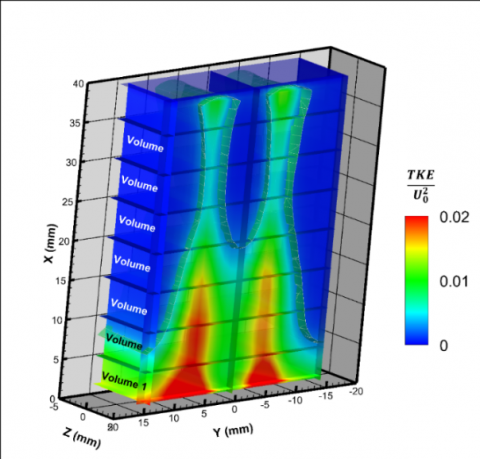Figure 3. Normalized TKE average field derived from Tomographic-PIV images

To obtain the $\frac{T K E}{U_{0}^{2} * \text {Volume}}$ in different zones of the flow, we chose to start with the volume, beginning with the impinged surface at $X=0$ and ending at $X=35 \mathrm{mm}$ from the nozzle exit in such a way that it contained vortices, as was shown in previous studies $ .$ The next volume was $5 \mathrm{mm}$ smaller, i.e. for $0<X<30 \mathrm{mm} .$ This procedure was applied until we obtained the last volume (Volume 1 in Figure 3 ) between $X=$ 0 and $X=5 m m .$ This choice was made to ensure that the region of impact in the volume of calculation was preserved in order to determine the influence of the extension of Volume 1 in the direction of the nozzle exit on TKE.

In Figure 4 we plotted the temporal evolution of $\frac{T K E}{U_{0}^{2} * \text {Volume}}$ eand its spectrum extracted from different sub-volumes of the flow as illustrated in Figure $3 .$ One can see that the temporal esignal of normalized TKE have higher amplitudes as the volume of interest become smaller. For example in Figure $4 . a$ (volume 7 which is the bigger one), the amplitude of normalized TKE reach a maximum of about $7.9 * 10^{-3}$ per $\mathrm{mm}^{3}$ when it reaches a maximum of about $4.25 * 10^{-2}$ per $\mathrm{mm}^{3}$ for volume 1 (the smaller volume). Actually, by excluding zones of low turbulent activity far from the wall of impact the relative TKE per unit of volume become higher and higher for high turbulent zones. One can also see in Figure 4 that the power spectra of $\frac{T K E}{U_{0}^{2} * \text {Volume}}$ for all volumes (volume 1 to $7 ;$ Figure 4 ) have the same main peak of frequency $\left(F_{0}=148 \mathrm{Hz}\right) .$ In Figure $4 . \mathrm{g},$ where volume 1 was considered (very close form the surface of impact), there was a harmonic frequency in the power spectrum due to the interaction with the slotted plate: $F_{1}=2 * F_{0}=296 \mathrm{Hz}$.

From these observations, we can assume that the main physical phenomenon, which is governing the fluctuations of the flow (and therefore the TKE production) between the jet exit and the plate, is the same because it is happening at the same frequency. The change of amplitudes arrives from the normalization by the volume. Indeed, a bigger volume do not contain more TKE because this latter is mainly produced near from the wall of impact. In order to strength this assumption, in Figure 5 we plotted the cross-correlations between the normalized TKE extracted from different volumes to verify theirs timing of happening.

## 4a.png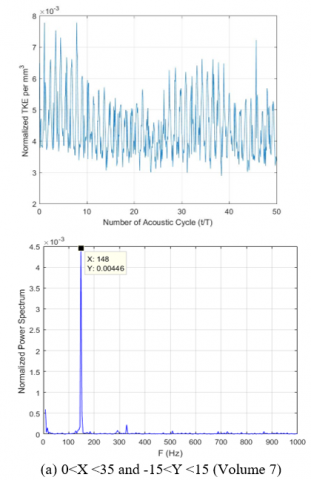## 4b.png## 4c.png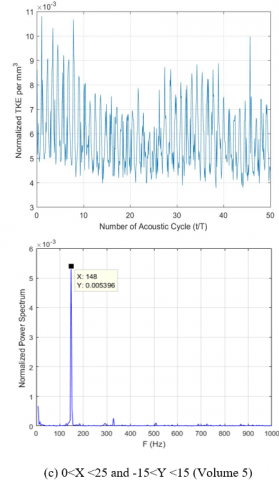## 4d.png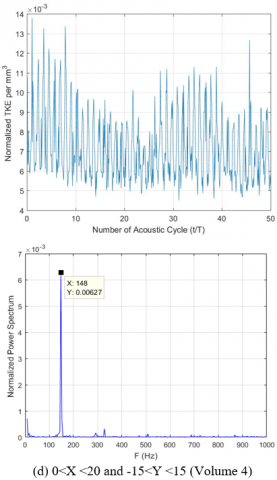## 4e.png## 4f.png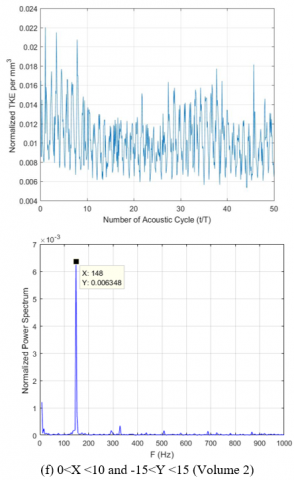## 4g.pngFigure 4. Spatio-temporal change and normalized power spectrum of normalized TKE per mm3. (a) 0<X <35 and -15<Y <15 (Volume 7), (b) 0<X <30 and -15<Y <15 (Volume 6), (c) 0<X <25 and -15<Y <15 (Volume 5), (d) 0<X <20 and -15<Y <15 (Volume 4), (e)0<X <15 and -15<Y <15 (Volume 3), (f)0<X <10 and -15<Y <15 (Volume 2) and (g) 0<X <5 and -15<Y <15 (Volume 1)

In Figure 5, one can see that the cross-correlations between the TKE signals from different volumes (volume 1 to volume 7, as in Figure 4) have a centered peak of correlation at t=0 what preserved the same phase for these signals. This means that these signals are synchronized. We can also observe a high coefficient of correlation at t=0s .

## 5a.png## 5b.png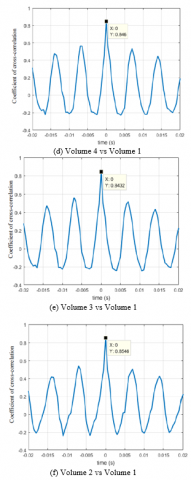Figure 5. Cross correlations between $\mathrm{TKE} /\left(\mathrm{U}_{0}^{2} * \text { Volume }\right)$ from different volumes. (a) Cross-correlation between $\mathrm{TKE} /\left(\mathrm{U}_{0}^{2} * \text { Volume }\right)$ obtained from Volume 7 and Volume 1, (b) Cross-correlation between $\mathrm{TKE} /\left(\mathrm{U}_{0}^{2} * \text { Volume }\right)$ obtained from Volume 6 and Volume 1, (c) Cross-correlation between $\mathrm{TKE} /\left(\mathrm{U}_{0}^{2} * \text { Volume }\right)$ obtained from Volume 5 and Volume 1, (d) Cross-correlation between $\mathrm{TKE} /\left(\mathrm{U}_{0}^{2} * \text { Volume }\right)$ obtained from Volume 4 and Volume 1, (e) Cross-correlation between $\mathrm{TKE} /\left(\mathrm{U}_{0}^{2} * \text { Volume }\right)$ obtained from Volume 3 and Volume 1, (f) Cross-correlation between $\mathrm{TKE} /\left(\mathrm{U}_{0}^{2} * \text { Volume }\right)$ obtained from Volume 2 and Volume 1

Therefore, TKE obtained from volume 1 could be considered as a reduced volume to represent the evolution of the TKE of the flow between the outlet of the jet and the impinged wall.

In Figure 6, the $\lambda_{2}$ criterion (defined as the second negative eigenvalue of the pressure tensor) was used in the same configuration to detect vortices. This criterion was introduced to detect the centre position of a rotary structure . Unlike vorticity, this criterion allows to distinguish between the shear and a rotational movement in the assumption that the centre of a vortex corresponds to a minimum of local pressure. This Figure shows that the vortices could be contained in a volume smaller than volume $1,$ as illustrated in Figure 7 a. since acoustic generation is triggered by the deformation of these vortices on the wall of impact, a new volume 1.1 will be tested, as shown in Figure 7 b $(0<$ $X<5 m m \text { and }-10 m m<Y<10 m m)$.

## 6.png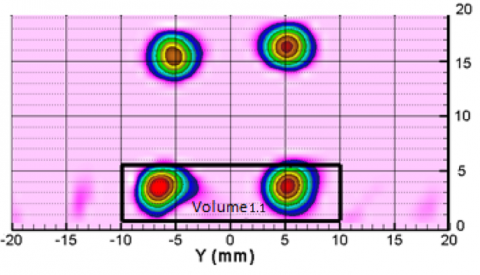Figure 6. Vortices using the λ2 criterion

In order to verify if volume 1.1 could replace volume 1 in representing the TKE evolution, in Figure 8 we plot the temporal change of the normalized TKE (Figure 8 a) and the cross-correlation between $\frac{T K E}{U_{0}^{2} \text { Volume }}$ from volume 1 and volume 1.1 (Figure 8 b). The cross-correlation between these two signals showed that they had the same phase (peak of correlation centered at $t=0 s$) with a high coefficient of correlation of 0.95.

## 7.png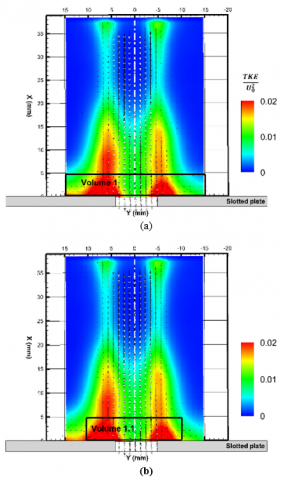Figure 7. Volume 1 and Volume 1.1

## 8.pngFigure 8. Temporal evolution of TKE for volume 1 and volume 1.1

One could suggest a volume of the jet (similar to volume 1.1 illustrated on Figure 6) that have a height of $0.5 * H$ and a length of $2 * H$ in order to analyze the evolution of the TKE in case of a rectangular jet impinging on a slotted plate.

4. Conclusions

TKE was considered in this study for a rectangular jet impinging on a slotted plate. A Reynolds number Re $=5435$ presenting self-sustained tones was investigated. The velocity field for a chosen volume was obtained from High Speed Tomographic-PIV images in order to calculate the TKE with respect to time and space. The aim of this study was to identify a reduced volume of the flow where TKE could be considered to investigate its changes. Based on the TKE spectrum and cross-correlations, it was found that a sub-volume of height equal to $0.5 * H,$ a length of $2 * H$ starting from the wall of impact was satisfactory to represent the TKE activity. This study is essential to continue the investigation on the interaction between the TKE field and the acoustic signal, which should be measured using a microphone in order to better understand the energy transfer between the aerodynamic and acoustic fields. This approach is important in the development of ways to control acoustic noise. It saves computing time and helps us in considering the active part of the jet that could be the bath of energy for the noise generation. Methods of decomposition (for example the Proper Orthogonal Decomposition and the Dynamic Mode Decompositions) could be also tested on the reduced volume in order to extract significant modes of the flow.

Acknowledgment

The authors wish to thank FEDER and the region of Nouvelle Aquitaine for its financial support.

Nomenclature
 B&K Brüel & Kjær f Frequency, Hz H Height of the convergent, m L Width of the convergent, m PIV Particle Image Velocimetry Re Reynolds Number T Period of the acoustic tones, s TKE Turbulent Kinetic Energy, j U Velocity in X direction, m.s-1 U0 Free stream velocity, m.s-1 u’ Fluctuation of velocity in X direction, m.s-1 Um Mean velocities in X direction, m.s-1 V Velocity in Y direction, m.s-1 v’ Fluctuation of velocity in Y direction, m.s-1 Vm Mean velocities in Y direction, m.s-1 W Velocity in Z direction, m.s-1 w’ Fluctuation of velocity in Z direction, m.s-1 Wm Mean velocities in Z direction, m.s-1 Greek symbols $\rho_{0}$ Density of the air, kg/m3 v Kinematic viscosity of air, m2.s-1 λ2 λ2 (eigenvalue)
References

 Abed, B.A., Beriache, M., Bettahar A. (2018). Experimental study of aero-thermal heat sink performances subjected to impinging air flow. International Journal of Heat and Technology, 36(4): 1310-1317. https://doi.org/10.18280/ijht.360420

 Vinod, P.D., Singh, S.N. (2017). Thermo-hydraulic performance analysis of jet plate solar air heater under cross flow condition. International Journal of Heat and Technology, 35(3): 603-610. https://doi.org/10.18280/ijht.350317

 Ho, C.M., Nosseir, N.S. (1981). Dynamics of an impinging jet. Part 1. The feedback phenomenon. Journal of Fluid Mechanics, 105: 119-142. https://doi.org/10.1017/S0022112081003133

 Ho, C.M., Nosseir, N.S. (1979). Large coherent structures in an impinging turbulent jet. Presented at the 2nd Symposium on Turbulent Shear Flows, 7: 26-31.

 Johnson, V.E., Chahine, G.L., Lindenmuth, W.T., Conn, A.F., Frederick, G.S., Giacchino, G.J. (1984). Cavitating and structured jets for mechanical bits to increase drilling rate-part I: Theory and concepts. Journal of Energy Resources Technology, 106(2): 282-288. https://doi.org/10.1115/1.3231053

 Genoux, F., Chahine, G.L. (1984). Simulation of the pressure field due to a submerged oscillating jet impacting on a solid wall. Journal of Fluids Engineering, 106(4): 491-495. https://doi.org/10.1115/1.3243156

 Assoum, H.H., El Hassan, M., Abed-Meraim, K., Sakout, A. (2014). The vortex dynamics and the self sustained tones in a plane jet impinging on a slotted plate. European Journal of Mechanics - B/Fluids, 48: 231-235. https://doi.org/10.1016/j.euromechflu.2014.06.008

 Assoum, H.H., Hassan, M.E., Abed-Meraïm, K., Martinuzzi, R., Sakout, A. (2013). Experimental analysis of the aero-acoustic coupling in a plane impinging jet on a slotted plate. Fluid Dynamics Research, 45(4): 045503. https://doi.org/10.1088/0169-5983/45/4/045503

 Hamdi, J., Assoum, H., Abed-Meraïm, K., Sakout, A. (2017). Volume reconstruction of an impinging jet obtained from stereoscopic-PIV data using POD. European Journal of Mechanics - B/Fluids, 67: 433-445. https://doi.org/10.1016/j.euromechflu.2017.09.001

 Hamdi, J., Assoum, H., Abed-Meraïm, K., Sakout, A. (2019). Analysis of the effect of the 3C kinematic field of a confined impinging jet on a slotted plate by stereoscopic PIV. European Journal of Mechanics - B/Fluids, 76: 243-258. https://doi.org/10.1016/j.euromechflu.2019.02.012

 Crow, S.C., Champagne, F.H. (1971). Orderly structure in jet turbulence. Journal of Fluid Mechanics, 48(3): 547-591. https://doi.org/10.1017/S0022112071001745

 Johnson, V.E., Lindenmuth, W.T., Conn, A.F., Frederick, G.S. (1981). Feasibility study of tuned-resonator, pulsating cavitating water jet for deep-hole drilling. Technical Report. https://doi.org/10.2172/6266875

 Chahine, G.L., Courbie`re, P. (1987). Noise and erosion of self-resonating cavitating jets. Journal of Fluids Engineering, 109(4): 429-435. https://doi.org/10.1115/1.3242684

 Powell, A. (1964). Theory of vortex sound. The Journal of the Acoustical Society of America, 36(1): 177-195. https://doi.org/10.1121/1.1918931

 Howe, M.S. (1975). Contributions to the theory of aerodynamic sound, with application to excess jet noise and the theory of the flute. Journal of Fluid Mechanics, 71(4): 625-673. https://doi.org/10.1017/S0022112075002777

 Toutiaei, S., Semaan, R., Naughton, J. (2010). Reynolds stress and turbulence kinetic energy balances in swirling jets. 48th AIAA Aerospace Sciences Meeting Including the New Horizons Forum and Aerospace Exposition, American Institute of Aeronautics and Astronautics. https://doi.org/10.2514/6.2010-105

 Lai, C.C.K., Socolofsky, S.A. (2019). Budgets of turbulent kinetic energy, Reynolds stresses, and dissipation in a turbulent round jet discharged into a stagnant ambient. Environmental Fluid Mechanics, 19(2): 349-377. https://doi.org/10.1007/s10652-018-9627-3

 Alekseenko, S.V., Bilsky, A.V., Dulin, V.M., Markovich, D.M. (2007). Experimental study of an impinging jet with different swirl rates. Revised and Extended Papers from the 5th Conference in Turbulence, Heat and Mass Transfer, 28(6): 1340-1359. https://doi.org/10.1016/j.ijheatfluidflow.2007.05.011

 Zeiny, E., Farhadi, M., Sedighi, K. (2017). Numerical investigation of the simultaneous influence of swirling flow and obstacles on plate in impinging jet. International Journal of Heat and Technology, 35(1): 59-66. https://doi.org/10.18280/ijht.350108

 Shekhar, C., Nishino, K. (2019). Turbulence energetics in an axisymmetric impinging jet flow. Physics of Fluids, 31(5): 055111. https://doi.org/10.1063/1.5086949

 Uddin, Naseem. (2008). Turbulence modeling of complex flows in CFD. OPUS - Online Publikationen der Universität Stuttgart. http://dx.doi.org/10.18419/opus-3781

 Assoum, H.H., Hamdi, J., Abed-Meraïm, K., El Hassan, M., Hammoud, A., Sakout, A. (2017). Experimental investigation the turbulent kinetic energy and the acoustic field in a rectangular jet impinging a slotted plate. Energy Procedia, 139: 398-403. https://doi.org/10.1016/j.egypro.2017.11.228

 Elsinga, G.E., Scarano, F., Wieneke, B., Van Oudheusden, B.W. (2006). Tomographic particle image velocimetry. Experiments in Fluids, 41(6): 933-947. https://doi.org/10.1007/s00348-006-0212-z

 Wieneke, B. (2008). Volume self-calibration for 3D particle image velocimetry. Experiments in Fluids, 45(4): 549-556. https://doi.org/10.1007/s00348-008-0521-5

 Assoum, H., Sakout, A., Abed-Meraim, K. (2014). Etude de la dynamique tourbillonnaire d’un jet plan heurtant une plaque fendue et le champ acoustique généré, CFA 2014, Poitiers, France

 Jeong, J., Hussain, F. (1995). On the identification of a vortex, Journal of Fluid Mechanics, 285: 69-94. https://doi.org/10.1017/S0022112095000462